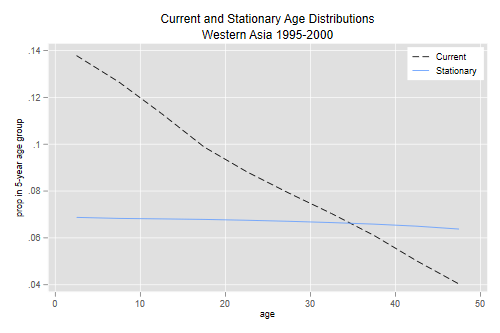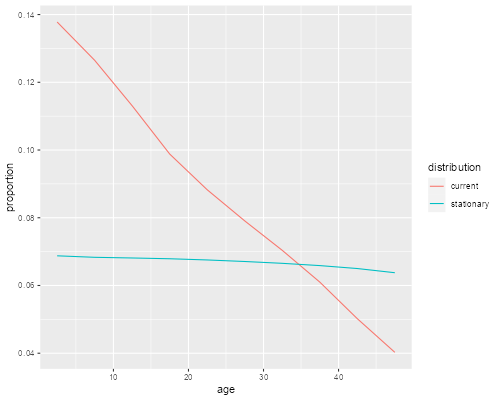Germán Rodríguez
Demographic Methods Princeton University## Population Momentum

This unit focuses on population momentum, the notion that for most of the world the population would continue to grow even if fertility dropped suddenly to replacement levels.

### Momentum in Western Asia

We turn to Box 7.3 in the textbook, which uses data from Western Asia in 1995-2000 to illustrate the Preston-Guillot calculations. We start by entering the female population, the survival ratios, and the maternity function, and then compute the NRR

```. clear

. mata:
───────────────────────────────────────────────── mata (type end to exit) ──────
:   N = (12013, 11027, 9856, 8614, 7694, 6893, 6135, 5318, 4376, 3510)'

:   L = (4.834, 4.803, 4.789, 4.773, 4.748, 4.716, 4.678, 4.631, 4.570, 4.483)'

:   m = (0, 0, 0, 0.043, 0.112, 0.112, 0.058, 0.029, 0.007, 0)'

:   nrr = sum (L :* m)

:   nrr
1.70282

: end
────────────────────────────────────────────────────────────────────────────────
```
```> N <- c(12013, 11027, 9856, 8614, 7694, 6893, 6135, 5318, 4376, 3510)
> L <- c(4.834, 4.803, 4.789, 4.773, 4.748, 4.716, 4.678, 4.631, 4.570, 4.483)
> m <- c(0, 0, 0, 0.043, 0.112, 0.112, 0.058, 0.029, 0.007, 0)
> nrr <- sum (L * m)
> nrr
 1.70282
```

We see that the net reproduction ratio is 1.703 daughters per woman. The population of Western Asia was growing fast at the turn of the century. What would have happened if fertility had dropped instantly to replacement level?

One way to answer the question would be to adjust the maternity function and do the projection. Here we will consider the alternatives.

### The Preston-Guillot Method

We first need to estimate the replacement-level maternity function, which we do by simply dividing the observed rates by the NRR. (Note that there are many other maternity functions that would work just as well, we follow tradition in assuming a proportionate decline.) We also need the mean age of the new maternity schedule.

```. mata:
───────────────────────────────────────────────── mata (type end to exit) ──────
:   ms = m/nrr

:   sum(L :* ms)
1

:   a = range(0,45,5) :+ 2.5

:   as = sum( a :* ms :* L)

:   as
26.60042753

: end
────────────────────────────────────────────────────────────────────────────────
```
```> ms <- m/nrr
> sum(L * ms)
 1
> a <- seq(0, 45,5) + 2.5
> as <- sum(a * ms * L)
> as
 26.60043
```

The mean age is 26.60, in agreement with the textbook. (Note that we computed just the numerator of the mean because the denominator is of course one, as we verified in the previous step.)

The only tricky step is calculation of the weight function, representing the expected number of births that will occur above each age divided by the sum of those births over all ages, which is, of course, the mean age at birth in the stationary population. (The analogy is integrating a survival curve to obtain expectation of life.)

The calculation requires a tail sum, which we obtain as a total sum minus the cumulative or running sume:

```. mata:
───────────────────────────────────────────────── mata (type end to exit) ──────
:   ba = ms :* L

:   w = 5 :* (ba:/2 + (sum(ba) :- runningsum(ba))):/as

:   w
1
┌───────────────┐
1 │  .1879669037  │
2 │  .1879669037  │
3 │  .1879669037  │
4 │  .1766391828  │
5 │  .1359612405  │
6 │  .0774586087  │
7 │  .0333310594  │
8 │  .0109435788  │
9 │  .0017656186  │
10 │            0  │
└───────────────┘

: end
────────────────────────────────────────────────────────────────────────────────
```
```> ba <- ms * L
> w <- 5 * (ba/2 + (sum(ba) - cumsum(ba)))/as
> w
 0.187966904 0.187966904 0.187966904 0.176639183 0.135961241 0.077458609
 0.033331059 0.010943579 0.001765619 0.000000000
```

These values are 5 times those published in the textbook, the reason will be revealed at the end of this section.

Now all we need to do is compute the ratio of the current to the stationary age distribution, multiply by the weights we just computed, and sum across ages (see Equation 7.21 in the textbook). In computing the age distributions we divide the numbers in each age group by the total number in all ages, not just 0-50, using the values given in Box 7.3, namely the population total for the N’s and life expectancy for the L’s. (If we had the data for all ages we could, of course, compute these ourselves.)

```. mata:
───────────────────────────────────────────────── mata (type end to exit) ──────
:   ca = N/87176

:   sa = L/70.3

:   sum( w :* ca :/ sa)
1.609443248

: end
────────────────────────────────────────────────────────────────────────────────
```
```> ca <- N/87176
> sa <- L/70.3
> sum( w * ca / sa)
 1.609443
```

And we discover that the female population will grow another 61.0%, even if rates dropped immediately to replacement level. A similar calculation with the male population and life table and the same weights shows that the male population would grow another 50.4%. The weighted average of these numbers is 56%. Finally we plot the current and stationary age distributions.

```. set obs 10
Number of observations (_N) was 0, now 10.

. mata:
───────────────────────────────────────────────── mata (type end to exit) ──────

: end
────────────────────────────────────────────────────────────────────────────────

. line ca sa a, lpat(dash solid) xtitle(age) ytitle(prop in 5-year age group) //
> /
>   title(Current and Stationary Age Distributions) ///
>   subtitle(Western Asia 1995-2000) ///
>   legend(order(1 "Current" 2 "Stationary") col(1) ring(0) pos(1))

. graph export momentum.png, width(500) replace
file momentum.png saved as PNG format
``````> library(ggplot2)
> d <- data.frame( age = c(a,a), proportion = c(ca, sa),
+   distribution = c(rep("current",length(a)),rep("stationary",length(a))))
> ggplot(d, aes(age, proportion, color = distribution)) + geom_line()
> ggsave("momentumr.png", width = 500/72, height = 400/72, dpi = 72)
```The reason for the substantial momentum should be clear from the nature of the age distributions.

Now for the missing multiplier. The textbook doesn’t multiply by 5 in computing the weights, but divides the nLx’s by 5, which produces the same end result. However, as explained on page 162, all three ingredients are distributions that sum to one. Mine do, whereas the weights in the text sum to 0.2, as does the implied stationary age distribution. These, of course, appear in the numerator and denominator of equation 7.21, so the factor of five cancels. To plot the age distributions, however, one needs to use nLx/e0, not (nLx/5)/e0.

### Keyfitz’s Approximation

The idea of population momentum originated with Keyfitz, and proved quite influential in policy circles. His formulation assumed that the population was stable at the outset and the reduction in rates was proportionally the same at all ages, but neither assumption is required in the previous development. Because the method is still popular, however, we apply it to Western Asia.

In the original 1971 paper, momentum is given by b e0(R-1)/(R r m), but the expression in common use is Frauhental’s approximation b e0/√R, where R is the NRR. So momentum is directly proportional to the birth rate and the expectation of life and inversely proportional to the square root of the net reproduction ratio.

For our example we already have the NRR and the mean age of childbearing, so we just need the birth rate to apply the formula

```. mata
───────────────────────────────────────────────── mata (type end to exit) ──────
:   cbr = sum(N :* m)/87176

:   cbr
.0291918647

:   cbr * 70.3 / sqrt(nrr)
1.57265258

: end
────────────────────────────────────────────────────────────────────────────────
```
```> cbr <- sum(N * m)/87176; cbr
 0.02919186
> cbr * 70.3 / sqrt(nrr)
 1.572653
```

We obtain a momentum of 1.57, which is not far from the more exact 1.61 computed above. Not bad for a simple calculation.

